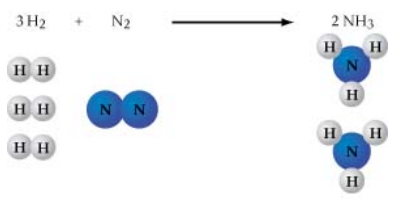# Problem: Notice that "SO4" appears in two different places in this chemical equation. SO42− is a polyatomic ion called "sulfate." What number should be placed in front of CaSO4 to give the same total number of sulfate ions on each side of the equation??CaSO4 + AlCl3 → CaCl2 + Al2(SO4)3Express your answer numerically as an integer.According to the law of conservation of mass, matter cannot be created or destroyed. Therefore, a chemical equation must show the same number of each kind of atom in the reactants as it does in the products. As shown in the figure, the balanced equation 3 H2 + N2 → 2 NH3 has 6 atoms of hydrogen and 2 atoms of nitrogen on each side of the arrow. One is often presented with unbalanced chemical equations for which one must supply the coefficients.The following equation is not balanced:CaSO4 + AlCl3 → CaCl2 + Al2(SO4)3

###### FREE Expert Solution
92% (498 ratings)View Complete Written Solution
###### Problem Details

Notice that "SO4" appears in two different places in this chemical equation. SO42− is a polyatomic ion called "sulfate." What number should be placed in front of CaSO4 to give the same total number of sulfate ions on each side of the equation?

?CaSO4 + AlCl3 → CaCl2 + Al2(SO4)3

According to the law of conservation of mass, matter cannot be created or destroyed. Therefore, a chemical equation must show the same number of each kind of atom in the reactants as it does in the products. As shown in the figure, the balanced equation 3 H2 + N2 → 2 NH3 has 6 atoms of hydrogen and 2 atoms of nitrogen on each side of the arrow. One is often presented with unbalanced chemical equations for which one must supply the coefficients.The following equation is not balanced:

CaSO4 + AlCl3 → CaCl2 + Al2(SO4)3